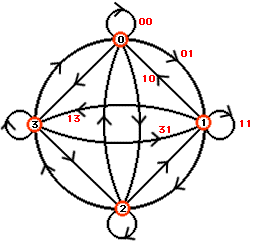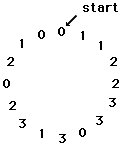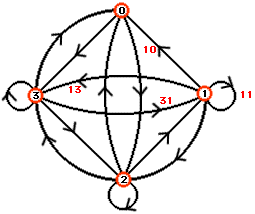Subject: Homework problem
Date: Sun, 18 Jan 1998 00:38:57 -0500

My name is Alexis. I'm a student in 8th grade and I'm taking Algebra I Honors. My question is: In a string of numbers, two adjacent digits are considered as a two-digit number. For instance, the string 11012 contains the numbers 10, 11, and 12. What is the number of digits in the smallest string that contains all of the two-digit numbers from 10-99? Please help and thank you for any assistance you can give me. (I would also appreciate it if you would explain how the problem was solved)

Hi Alexis,

Let me answer a somewhat simpler version of your problem that will show how to answer the question you gave us with a string of 99 digits.
Suppose that we only had the digits 0,1,2,3 to work with instead of 0,1,2,...,9. Further, suppose we were asked to find a string of these four digits that is as short as possible and also each of the 12 numbers 10,11,12,13,20,21,22,23,30,31,32, and 33 appear. (You should see the analogy with your question which asks for 10,11,...,19,...90,91,...,99 to appear.)

 Look at the diagram, it is called a directed graph. It has a vertex for each of the four digits 0,1,2,3 and a directed edge between each vertex and each other vertex (these are like one-way streets joining four towns). We also allow a directed loop at each vertex, for example from vertex 1 back to itself. In all there are 4x4=16 directed edges. We can name each edge by its ends, for example the edge from 0 to 0 is called 00, from 0 to 1 is called 01, from 1 to 0 is called 10, etc.  Suppose that you can start at any vertex, say vertex 0, and walk around the diagram using all the directed edges (you must not go the wrong way on a one-way street) exactly once and end up where you started. (Such a journey is called an Euler circuit after the 18th century Swiss mathematician Leonard Euler.)When we take such a journey we write down, in order, the names of the vertices we visit. One such journey is described in the diagram on the left. Look at the names of the "streets" we used: 01, 11, 12, 22, 23, 33, 30, 03, 31, 13, 32, 20, 02, 21, 10, 00. All sixteen "streets", or two digit numbers appear including 00, 01, 02, and 03 which we don't need. If we throw away our starting point, 0, we are left with a string 112233031320210 which does the job for us in 15=4x4-1 digits.  So, can we do it with less than 15 digits? The diagram on the right is the directed graph that remains when the edges 00 and 01 are removed and the path 112233031320210 represents a walk around the diagram using all the directed edges exactly once. Can you see why such a walk is impossible if you remove either of the edges 02 and 03 also?To answer your question we need 10 vertices labelled 0, 1, 2,...,9 and 100 directed edges, then an argument similar to the one above leads to a string of length 10x10-1=99.

Cheers,
Penny

Go to Math Central